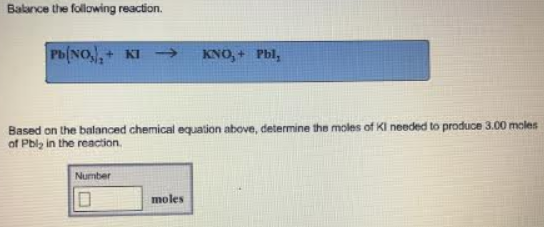# Problem: Balance the following reaction. Pb(NO3)3 + KI → KNO3 +PbI2Based on the balanced chemical equation above, determine the moles of KI needed to produce 3.00 moles of Pbl2 in the reaction.

###### FREE Expert Solution
91% (374 ratings)###### Problem Details

Balance the following reaction.

Pb(NO3)3 + KI → KNO3 +PbI2

Based on the balanced chemical equation above, determine the moles of KI needed to produce 3.00 moles of Pbl2 in the reaction.Frequently Asked Questions

What scientific concept do you need to know in order to solve this problem?

Our tutors have indicated that to solve this problem you will need to apply the Stoichiometry concept. You can view video lessons to learn Stoichiometry. Or if you need more Stoichiometry practice, you can also practice Stoichiometry practice problems.